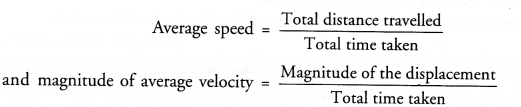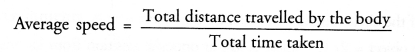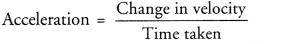# HOTS Questions for Class 9 Science Chapter 8 Motion

## HOTS Questions for Class 9 Science Chapter 8 Motion

These Solutions are part of HOTS Questions for Class 9 Science. Here we have given HOTS Questions for Class 9 Science Chapter 8 Motion

Question 1.
Under what condition, the average speed is equal to the magnitude of the average velocity. Justify your answer.
(CBSE 2010, 2012)Now, the average speed = magnitude of average velocity if the total distance travelled by an object in a given time interval is equal to the magnitude of the displacement of the object in the same time interval. This is possible, when an object moves in one direction along a straight line. Thus, average speed is equal to the magnitude of the average velocity if an object moves in one direction along a straight line.

More Resources

Question 2.
A body can have zero average velocity but not zero average speed. Explain. (CBSE 2013)

Or

Give an example of a motion in which average velocity is zero, but the average speed is not zero. (CBSE 2015)Since, the total distance travelled by a moving body increases with the time, so average speed of a moving body can not be zero.Since total displacement of a moving body can be zero, so the average velocity of a moving body can be zero.
Example. When an athlete completes one round in a circular track in the given time interval, then his total displacement is zero but total distance travelled is equal to the length of the circular track. Hence, his average velocity is zero but his average speed is not zero.

Question 3.
An object P is moving with a constant velocity for 5 minutes. Another object Q is moving with changing velocity for 5 minutes. Out of these two objects, which one has acceleration. Explain. (CBSE 2012)
Change in velocitySince, the velocity of object P is not changing or change in velocity of the object is zero, therefore, object P has no acceleration. On the other hand, there is change in velocity of the object Q, so it has acceleration.

Question 4.
Can an object be accelerated if it is moving with constant speed ? Justify your answer with an example.

Or

Can a body have constant speed and still be accelerating ? Give an example. (CBSE 2010)

Or

Explain how is it possible for an object to move with a constant speed but with uniform acceleration.
(CBSE 2012)